# How to Calculate Z Score in Excel

In Excel, the z score is a statistical measure that represents the number of standard deviations, that a data point is from the mean of a data set. To calculate the z score in Excel, you can use the Z.TEST function. We get it, it’s confusing, especially if you’re not familiar with math but don’t worry, we’ll get deep into calculating z score in excel. Keep reading to find out how to calculate z score in excel and also to find out about inverse z score excel.## How to Calculate Z Score in Excel

Here, you will get to know the ways to calculate Z score in Microsoft Excel.

### What is Z Score in Excel?

z score is a statistical measure, which represents the number of standard deviations. It is calculated by subtracting the mean of the data set from the value of the data point and then dividing that result by the standard deviation of the data set. The z score can be used to determine whether a value is typical or unusual for the data set. It can also be used to compare data from different data sets by standardizing the data.

You can use the Z.TEST function in Excel to calculate the z score for a specific data point, given a range of data. This can be useful for identifying outliers or unusual values in a data set.

It is calculated using the following formula:

z-score = (x – μ) / σ

where:

• x is the value of the data point.
• μ is the mean of the data.
• σ is the standard deviation of the data.

Also Read: How to Interpret Z Score

### How to Calculate Z Score in Excel

For calculating z score in excel, you can use the Standardized function. Let us see, with an example:

Suppose this is your data and you want to find the z score.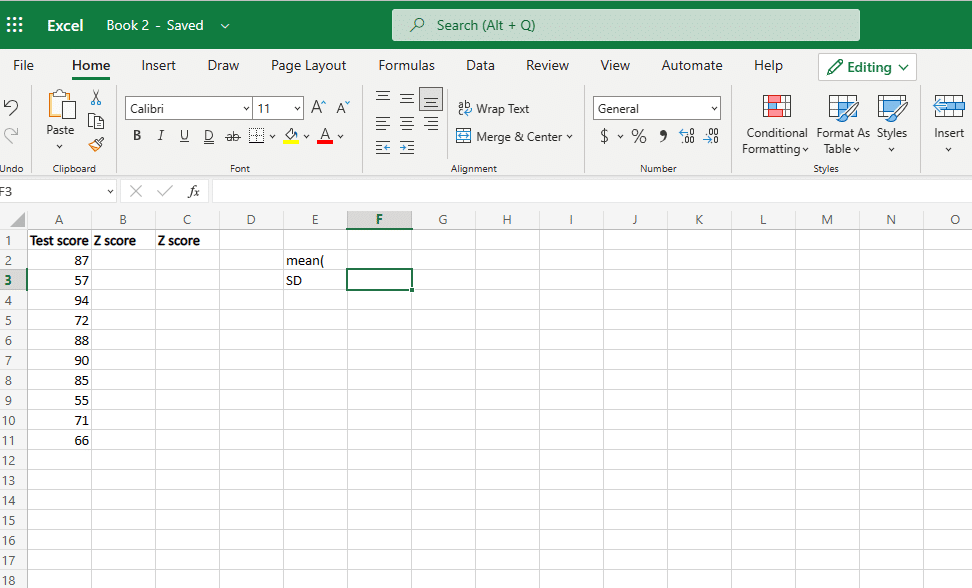Now, follow the steps to find the z score, but first, you need to find the mean and standard deviation.

In order to calculate your data’s mean and standard deviation. Follow the steps mentioned below.

1. Click on the new cell and type in equal’s average.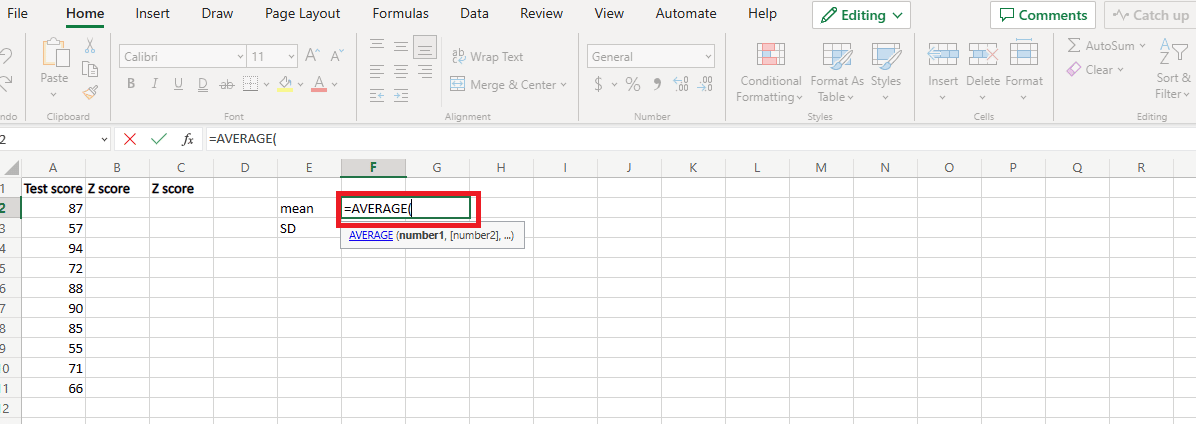2. Click and drag on the whole data set and close the bracket.3. Press the Enter key, and you’ll get your mean value.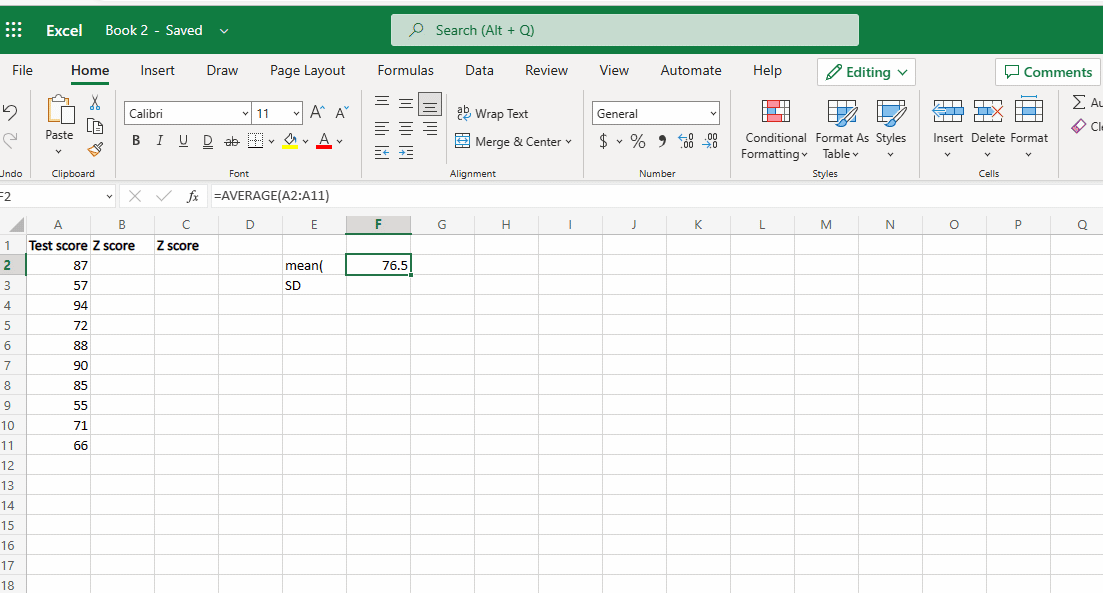Now, to calculate the Standard deviation

1. Type equals STDEV open bracket and again select the whole data.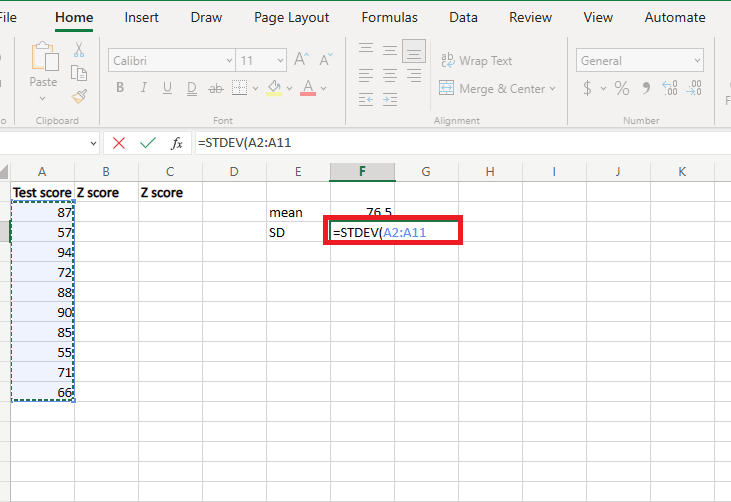2. Close the bracket and press the Enter key.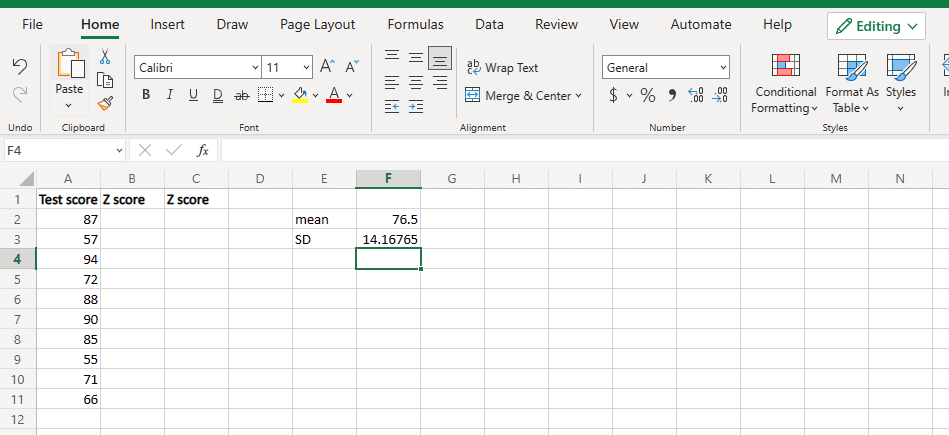So, here you have your standard deviation

Now, let’s calculate the z score for the first cell

1. Click on the new cell and type in equals, the data point, which is 87 in the example minus mean. Put them into brackets, so it will be (A2-F2) here divided by standard deviation which is F3.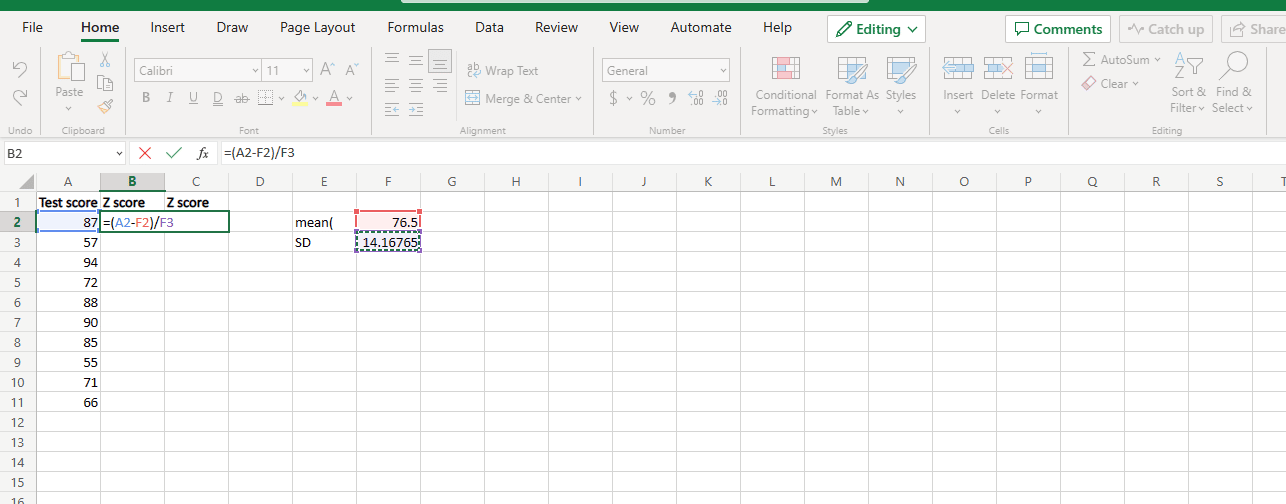2. Press the Enter key, to get the z score.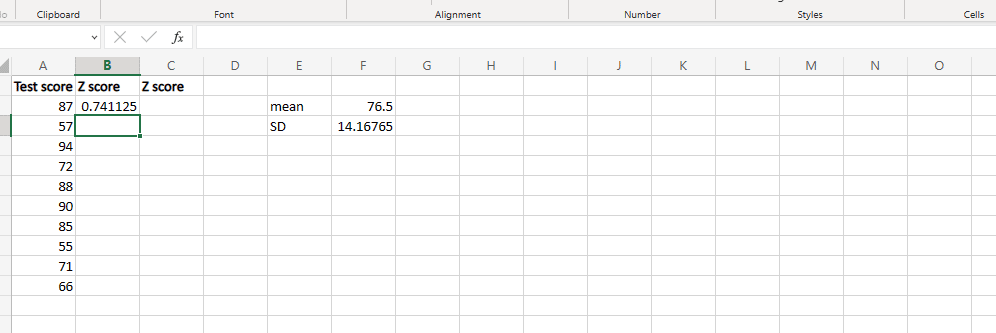3. Now, to repeat the same for all the values, add the \$ sign in the formula (A2-\$F\$2)/\$F\$3 and then simply drag down and click ctrl + D.

Note: Adding the dollar prefix helps to keep these two cells constant when finding the value for other cells.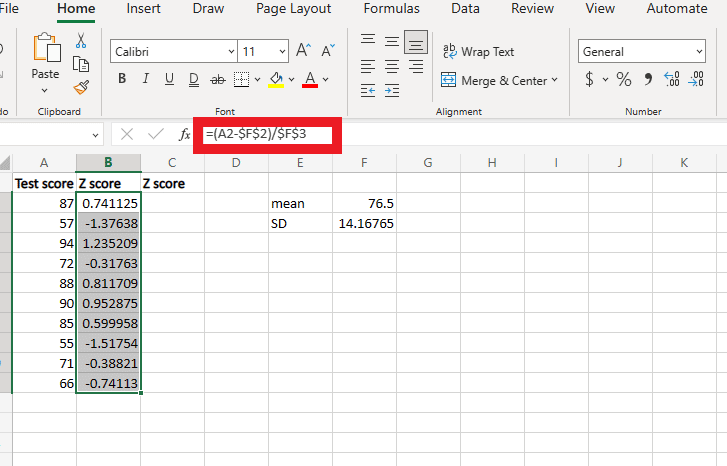Now, you have the z score value for each cell, hope this helped to learn how to calculate z score in excel.

Also Read: Swap Columns or Rows in Excel

### How to Interpret Z Scores in Excel

A value’s standard deviation from a data set’s mean is expressed as a z-score. The Z.TEST function in Excel can be used to determine a value’s z-score for a given data set.

To interpret the z-score, you can use a standard normal table (also known as a z-score table) to find the corresponding probability. For example, a z-score of 1.96 corresponds to a probability of approximately 0.975, which means that the value is within the upper 2.5% of the data. Conversely, a z-score of -1.96 corresponds to a probability of approximately 0.025, which means that the value is within the lower 2.5% of the data.

You can also use the z score table excel to determine whether a value is an outlier in the data set. A value with a z-score that is much higher or lower than the other values in the data set may be considered an outlier. For example, you might consider values with z-scores greater than 3 or less than -3 to be outliers. It’s important to keep in mind that the interpretation of a z-score depends on the context in which it is used.

Also Read: Fix Excel Slow to Open in Windows 10

### NORM.S. INV Function in Excel

NORM.S.INV is a function in Microsoft Excel that returns the inverse z score excel of the standard normal cumulative distribution. The standard normal cumulative distribution is a function that gives the probability that a random variable, drawn from a normal distribution with mean 0 and standard deviation 1, is less than or equal to a given value.

For example, if you want to find the value that corresponds to a probability of 0.9, you would use the following formula:

=NORM.S.INV (0.9)

The inverse z score excel is useful for working with the standard normal distribution and the Z table. Inverse Z score Excel allows you to find the value that corresponds to a given probability, which can be useful for statistical tests and procedures that involve the standard normal distribution.

Q1. What does z-score tell you?

Ans. A z-score, also known as a standard score, tells you how many standard deviations a value is from the mean of a data set. A z-score can be positive or negative, depending on whether the value is above or below the mean.

Q2. Why is z-score so important?

Ans. z scores are important because they allow you to compare values from different data sets by standardizing them. Because the z-score expresses the value in terms of standard deviations from the mean, you can compare values that may have different units or scales. z scores are also useful for identifying outliers in a data set.

Q3. Why is it called z-score?

Ans. The z-score is called a z-score because it is based on the standard normal distribution, which is a normal distribution with a mean of 0 and a standard deviation of 1. The letter z is often used to denote a standard normal random variable.

Q4. What is a good z-score value?

Ans. It is not meaningful to talk about a good or bad z-score value in general, because the interpretation of a z-score depends on the context in which it is used.

Q5. Where are z scores used?

Ans. z scores are used in a wide variety of fields and applications. z scores are often used to evaluate the performance of students relative to their peers. For example, a teacher may use z-scores to determine which students are performing above or below the average for their grade level.

Q6. What is the z table in Excel?

Ans. The z score table excel, also known as the standard normal table, is a table of values for the standard normal distribution.

Recommended:

We hope that this article was helpful in making you understand how to calculate z score in excel. Please go through the article and let us know if you have any doubts or queries in the comments section below.

#### Fix Office 365 Error 70003: Your Organization Has Deleted This Device

Jassweb always keeps its services up-to-date with the latest trends in the market, providing its customers all over the world with high-end and easily extensible internet, intranet, and extranet products.